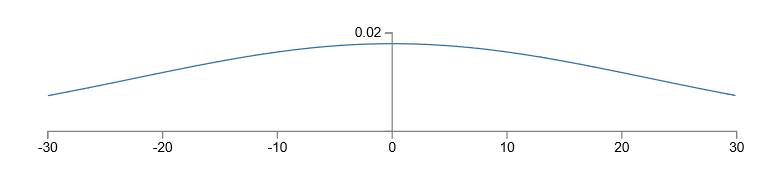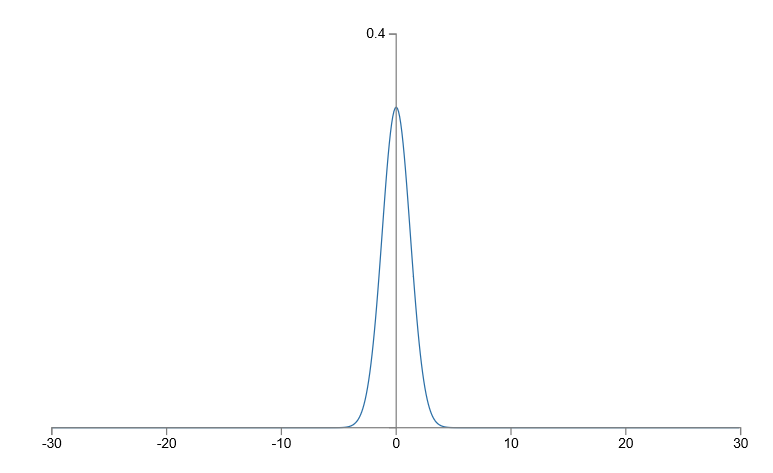95% 的识别率看起来很高了，但还有不少提升空间。本篇文章将介绍多种优化方法。

## 交叉熵代价函数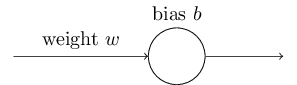\begin{eqnarray}
C = \frac{(y-a)^2}{2},
\label{54}
\end{eqnarray}

\begin{eqnarray}
\frac{\partial C}{\partial w} & = & (a-y)\sigma’(z) x = a \sigma’(z) \label{55}\\
\frac{\partial C}{\partial b} & = & (a-y)\sigma’(z) = a \sigma’(z),
\label{56}
\end{eqnarray}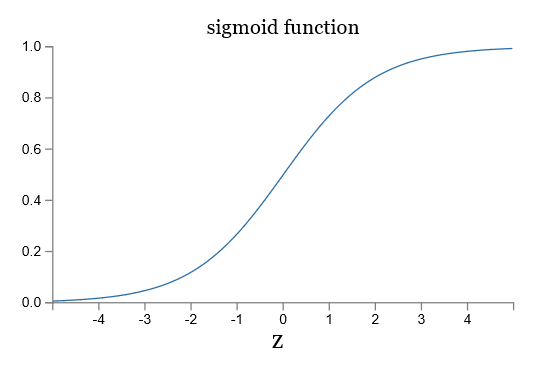### 介绍交叉熵代价函数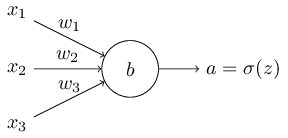\begin{eqnarray}
C = -\frac{1}{n} \sum_x \left[y \ln a + (1-y ) \ln (1-a) \right],
\label{57}
\end{eqnarray}

\begin{eqnarray}
\frac{\partial C}{\partial w_j} & = & -\frac{1}{n} \sum_x \left(
\frac{y }{\sigma(z)} -\frac{(1-y)}{1-\sigma(z)} \right)
\frac{\partial \sigma}{\partial w_j} \label{58}\\
& = & -\frac{1}{n} \sum_x \left(
\frac{y}{\sigma(z)}
-\frac{(1-y)}{1-\sigma(z)} \right)\sigma’(z) x_j.
\label{59}
\end{eqnarray}

\begin{eqnarray}
\frac{\partial C}{\partial w_j} & = & \frac{1}{n}
\sum_x \frac{\sigma’(z) x_j}{\sigma(z) (1-\sigma(z))}
(\sigma(z)-y).
\label{60}
\end{eqnarray}

\begin{eqnarray}
\frac{\partial C}{\partial w_j} = \frac{1}{n} \sum_x x_j(\sigma(z)-y).
\label{61}
\end{eqnarray}

\begin{eqnarray}
\frac{\partial C}{\partial b} = \frac{1}{n} \sum_x (\sigma(z)-y).
\label{62}
\end{eqnarray}

\begin{eqnarray} C = -\frac{1}{n} \sum_x
\sum_j \left[y_j \ln a^L_j + (1-y_j) \ln (1-a^L_j) \right].
\label{63}
\end{eqnarray}

### 柔性最大值传输 softmax

\begin{eqnarray}
a^L_j = \frac{e^{z^L_j}}{\sum_k e^{z^L_k}},
\label{78}
\end{eqnarray}

\begin{eqnarray}
\sum_j a^L_j & = & \frac{\sum_j e^{z^L_j}}{\sum_k e^{z^L_k}} = 1.
\label{79}
\end{eqnarray}

[0.9, 0.3, 0.4, 0.1, 0.0, 0.4, 0.0, 0.0, 0.0, 0.1]


## 过拟合和正则化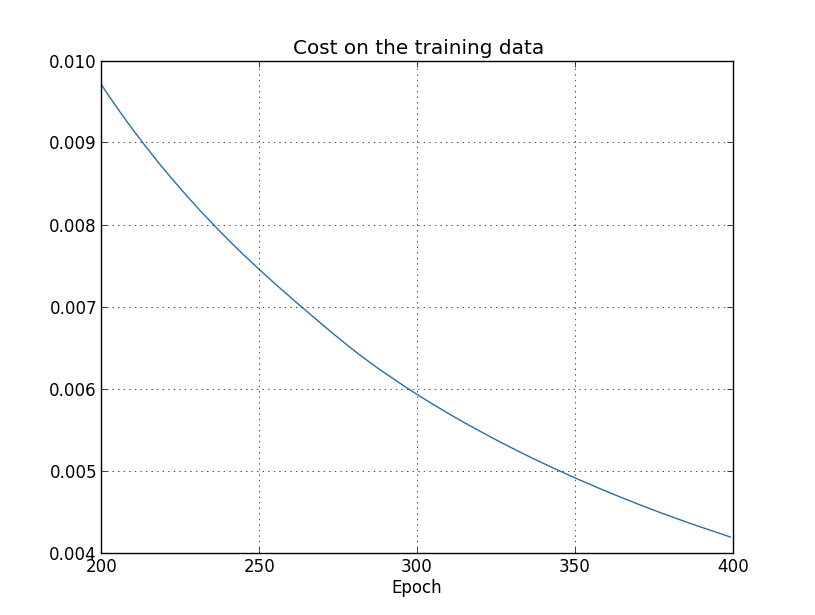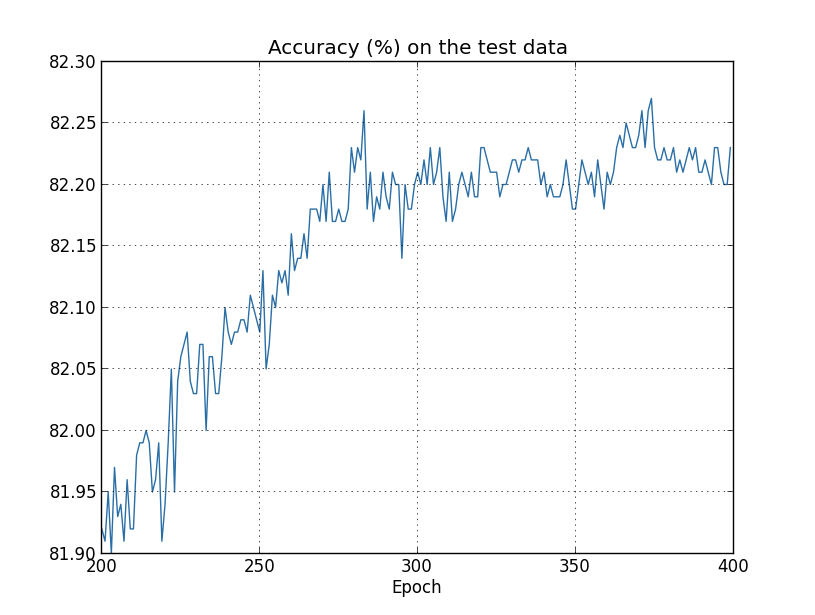280 个 epoch 之后，识别率处于波动稳定状态，且远低于之前达到的 95% 识别率。训练数据的交叉熵和测试集的实际结果截然不同，出现了费米担心的问题。可以说，280 个 epoch 之后的学习完全无用，标准说法是过拟合 overfitting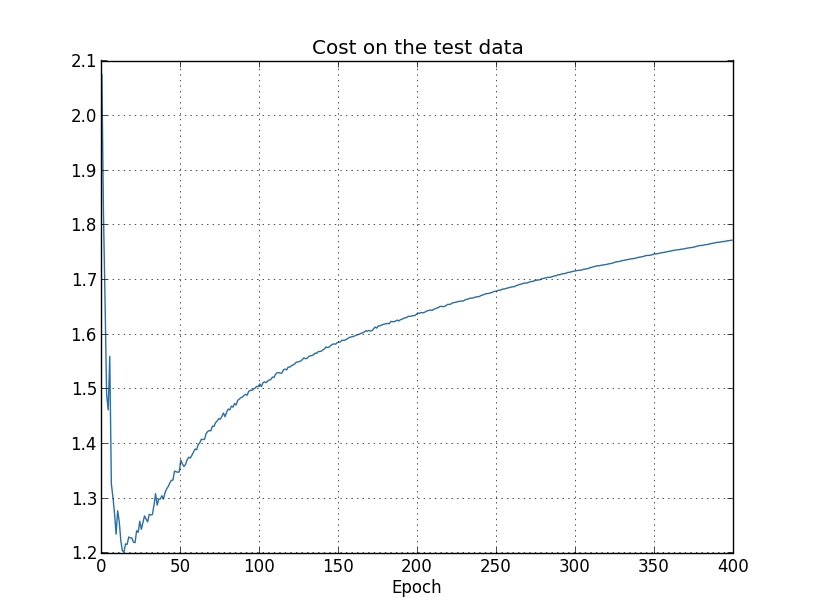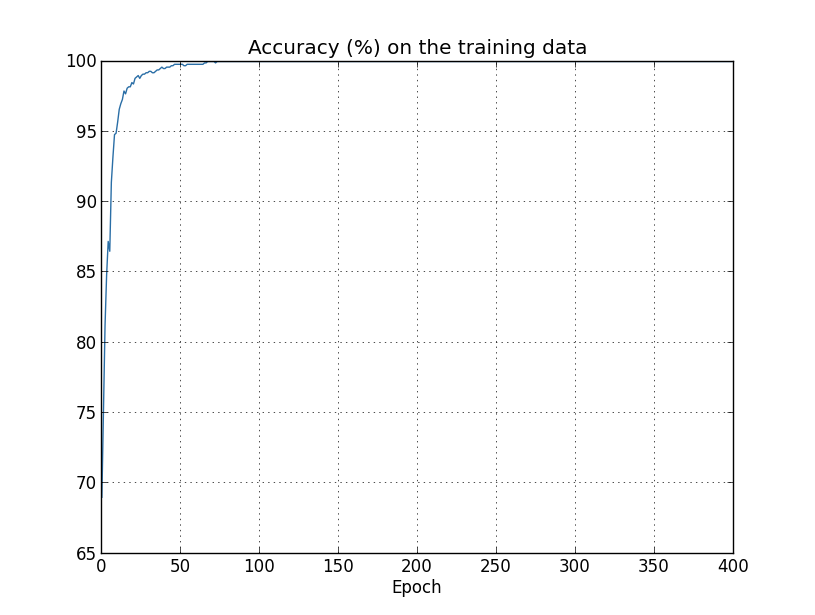training_data, validation_data, test_data = mnist_loader.load_data_wrapper()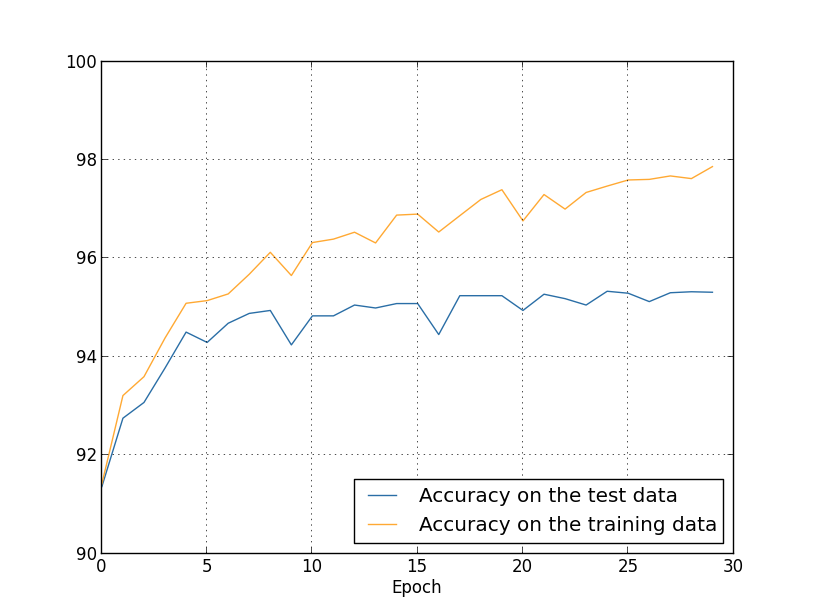### 正则化 Regularization

\begin{eqnarray}
C = -\frac{1}{n} \sum_{xj} \left[ y_j \ln a^L_j+(1-y_j) \ln
(1-a^L_j)\right] + \frac{\lambda}{2n} \sum_w w^2.
\label{85}
\end{eqnarray}

\begin{eqnarray}
C = \frac{1}{2n} \sum_x |y-a^L|^2 +
\frac{\lambda}{2n} \sum_w w^2.
\label{86}
\end{eqnarray}

\begin{eqnarray}
C = C_0 + \frac{\lambda}{2n}
\sum_w w^2,
\label{87}
\end{eqnarray}

\begin{eqnarray}
\frac{\partial C}{\partial w} & = & \frac{\partial C_0}{\partial w} +
\frac{\lambda}{n} w \label{88}\\
\frac{\partial C}{\partial b} & = & \frac{\partial C_0}{\partial b}.
\label{89}
\end{eqnarray}

$\partial C_0 / \partial w$ 和 $\partial C_0 / \partial b$ 仍让可以用上一篇的反向传播算法求得。对偏移的偏导数并没有改变，所以据梯度下降法学习规则仍为：

\begin{eqnarray}
b & \rightarrow & b -\eta \frac{\partial C_0}{\partial b}.
\label{90}
\end{eqnarray}

\begin{eqnarray}
w & \rightarrow & w-\eta \frac{\partial C_0}{\partial
w}-\frac{\eta \lambda}{n} w \label{91}\\
& = & \left(1-\frac{\eta \lambda}{n}\right) w -\eta \frac{\partial
C_0}{\partial w}.
\label{92}
\end{eqnarray}

\begin{eqnarray}
w \rightarrow \left(1-\frac{\eta \lambda}{n}\right) w -\frac{\eta}{m}
\sum_x \frac{\partial C_x}{\partial w},
\label{93}
\end{eqnarray}

\begin{eqnarray}
b \rightarrow b - \frac{\eta}{m} \sum_x \frac{\partial C_x}{\partial b},
\label{94}
\end{eqnarray}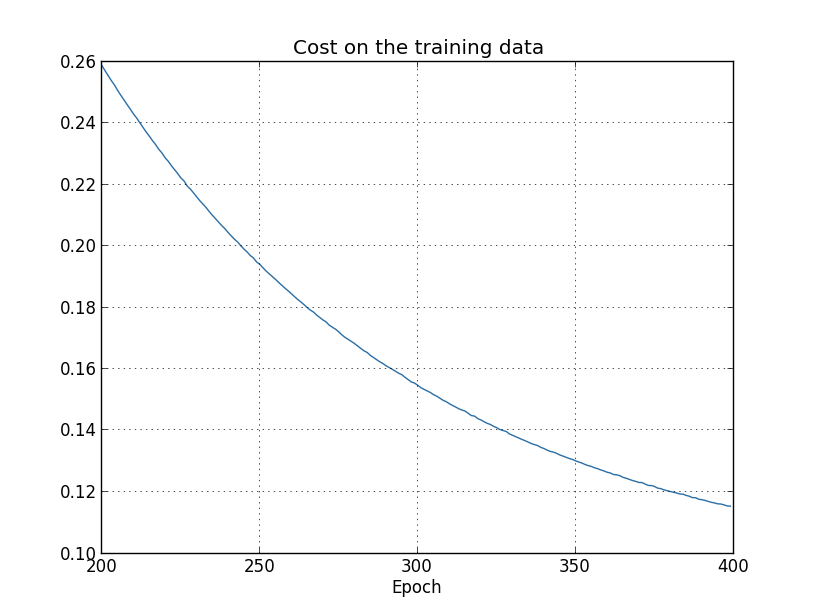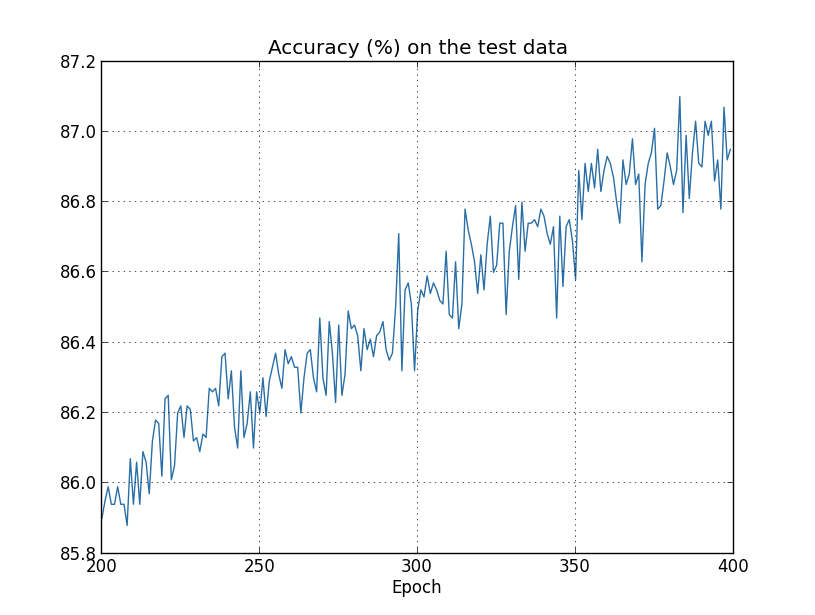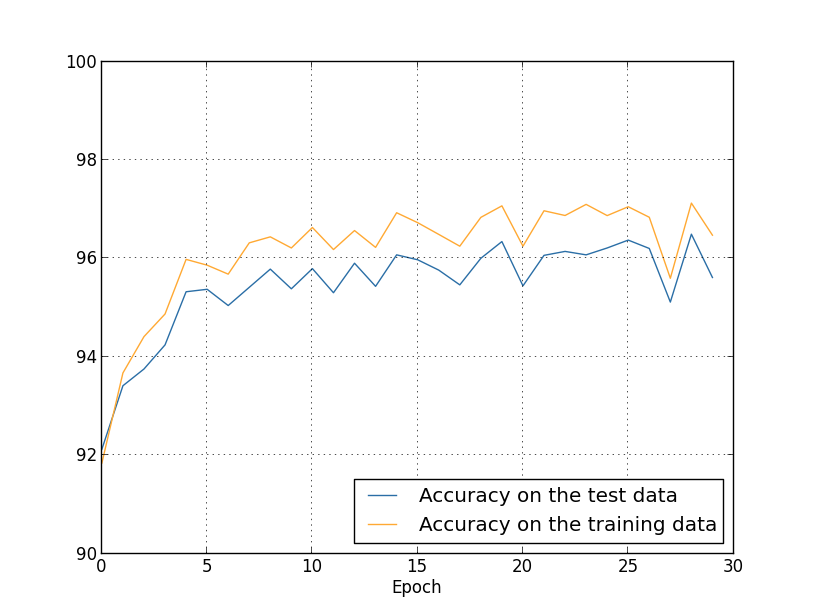### 为什么正则化能抑制过拟合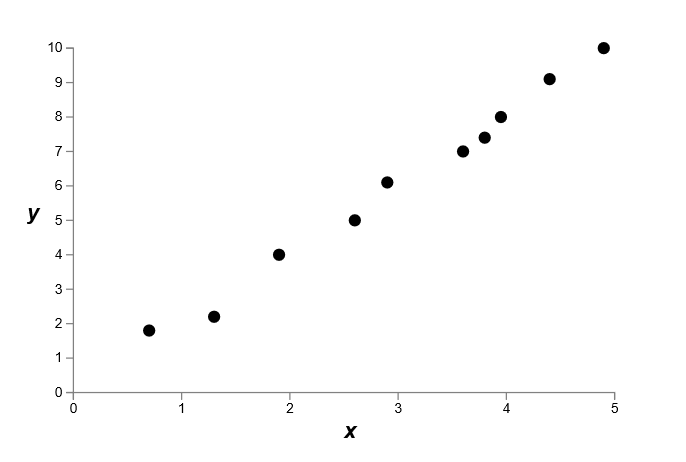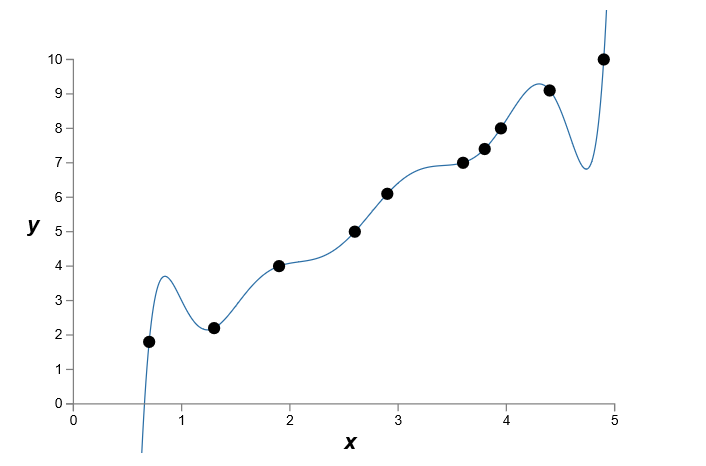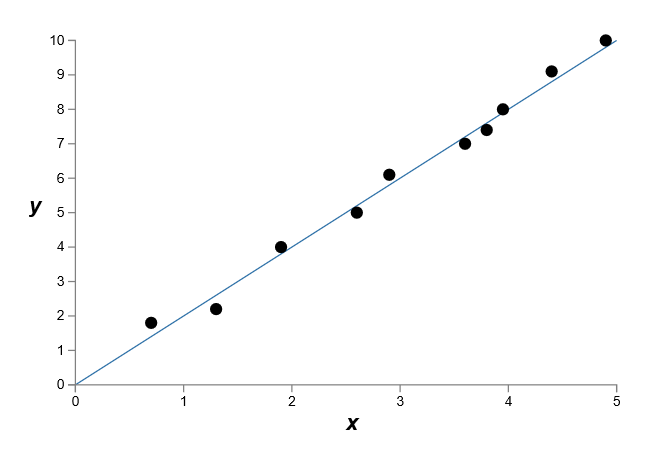### 其他抑制过拟合的方法

$L_1$ 正则化，即换一个正则函数。

dropout：学习过程中随机删去一些神经元。

## 改进权重初始化